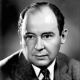# Group Theory 13: Finitely Generated Abelian Groups

The group $\mathbb{Z}\times\mathbb{Z}_2$ is generated by $(1,0)$ and $(0,1)$. In general, the direct product of $n$ cyclic groups, each of which is either $\mathbb{Z}$ or $\mathbb{Z}_m$ is generated by $(1,0,\cdots,0)$, $(0,1,0,\cdots,0)$, $\cdots$, $(0,0,\cdots,0,1)$. Such a direct product may be generated by fewer elements. For example, $\mathbb{Z}_3\times\mathbb{Z}_4\times\mathbb{Z}_{35}$ is generated by the single element $(1,1,1)$ i.e. it is a cyclic group. Conversely, we have the following theorem holds.

Theorem. [Fundamental Theorem of Finitely Generated Abelian Groups] Every finitely generated abelian group $G$ is isomorphic to a direct product of cyclic groups in the form
$$\mathbb{Z}_{p_1^{r_1}}\times\mathbb{Z}_{p_2^{r_2}}\times\cdots\times\mathbb{Z}_{p_n^{r_n}}\times\mathbb{Z}\times\mathbb{Z}\times\cdots\times\mathbb{Z},$$where the $p_i$ are primes, not necessarily distinct and the $r_i$ are positive integers. The direct product is unique except for a possible rearrangement of the factors. The number of factors $\mathbb{Z}$ is called the Betti number of $G$.

Example. Find all abelian groups, up to isomorphism, of order 360.

Solution. $360=2^3\cdot 3^2\cdot 5$, so all abelian groups, up to isomorphism, of order 360 are
\begin{align*}
\mathbb{Z}_2\times\mathbb{Z}_2\times\mathbb{Z}_2\times\mathbb{Z}_3\times\mathbb{Z}_3\times\mathbb{Z}_5,\\
\mathbb{Z}_2\times\mathbb{Z}_4\times\mathbb{Z}_3\times\mathbb{Z}_3\times\mathbb{Z}_5,\\
\mathbb{Z}_2\times\mathbb{Z}_2\times\mathbb{Z}_2\times\mathbb{Z}_9\times\mathbb{Z}_5,\\
\mathbb{Z}_2\times\mathbb{Z}_4\times\mathbb{Z}_9\times\mathbb{Z}_5,\\
\mathbb{Z}_8\times\mathbb{Z}_3\times\mathbb{Z}_3\times\times\mathbb{Z}_5,\\
\mathbb{Z}_8\times\mathbb{Z}_9\times\mathbb{Z}_5.
\end{align*}

Definition. A group $G$ is decomposable if it is isomorphic to a direct product of two proper nontrivial subgroups. Otherwise $G$ is indecomposable.

Theorem. The finite indecomposable abelian groups are exactly the cyclic groups with order a power of a prime.

Proof. If $G$ is a finite indecomposable abelian group, then by Fundamental Theorem of Finitely Generated Abelian Groups, $G$ is isomorphic to a direct product of cyclic groups of prime power order.Since $G$ is indecomposable, the direct product must consist of just one cyclic group of a prime power order. conversely, let $p$ be a prime. Then $\mathbb{Z}_{p^r}$ is indecomposable. If it were isomorphic to $\mathbb{Z}_{p^i}\times\mathbb{Z}_{p^j}$ where $i+j=r$, then every element has an order at most $p^{\max(i,j)}<p^r$.

Theorem. If $m$ divides the order of a finite abelian group $G$, then $G$ has a subgroup of order $m$.

Proof. Since $G$ is a finite abelian group,
$$G\cong\mathbb{Z}_{p_1^{r_1}}\times\mathbb{Z}_{p_2^{r_2}}\times\cdots\times\mathbb{Z}_{p_n^{r_n}}.$$Since $p_1^{r_1}p_2^{r_2}\cdots p_n^{r_n}=|G|$, $m$ must be of the form $p_1^{s_1}p_2^{s_2}\cdots p_n^{s_n}$, where $0\leq s_i\leq r_i$. For each $1\leq i\leq n$, $p_i^{r_i-s_i}$ generates a cyclic group of order$$\frac{p_i^{r_i}}{(p_i^{r_i},p_i^{r_i-s_i})}=\frac{p_i^{r_i}}{p_i^{r_i-s_i}}=p_i^{s_i}.$$So, $p_i^{r_i-s_i}$ generates a cyclic subgroup of $\mathbb{Z}_{p_i^{r_i}}$ of order $p_i^{s_i}$. Therefore,$$\langle p_1^{r_1-s_1}\rangle\times\langle p_2^{r_2-s_2}\rangle\times\cdots\times\langle p_n^{r_n-s_n}\rangle$$
is a subgroup of $G$ of order $m=p_1^{s_1}p_2^{s_2}\cdots p_n^{s_n}$.

Theorem. If $m$ is a square free integer i.e. if $m$ is not divisible by the square of any prime, then every abelian group of order $m$ is cyclic.

Proof. Let $G$ be an abelian group of square free order $m$. Then
$$G\cong\mathbb{Z}_{p_1^{r_1}}\times\mathbb{Z}_{p_2^{r_2}}\times\cdots\times\mathbb{Z}_{p_n^{r_n}},$$
where $m=p_1^{r_1}p_2^{r_2}\cdots p_n^{r_n}$. Since $m$ is square free, $r_i=1$, $i=1,\cdots,n$ and the $p_i$ are distinct. Hence, $G$ is isomorphic to $\mathbb{Z}_{p_1p_2\cdots p_n}$ i.e. it is cyclic.

## 2 thoughts on “Group Theory 13: Finitely Generated Abelian Groups”

1.Kade

there is a trivial typo, where should be 360=2^3∙3^2∙5

1.lee Post author